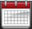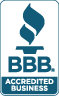713.589.2655
Houston, Texas

210.568.3904
San Antonio, Texas

 Course LinksSchedulesResourcesMicrosoft® Office Excel Level 4: Advanced FunctionsTraining Course Content Course Summary: Excel is one of the most powerful applications ever created. Take your skills and knowledge beyond the basic and intermediate level functions. Learn to harness the power that Excel offers by using more advanced formula techniques. Once you've worked with these functions, you'll be able to make Excel do some pretty amazing things. In this course we'll cover up to 40 Excel functions in a single day! Course Prerequisite: Due to the content, this course is fast paced. You should be very comfortable with the use of functions in Excel prior to enrolling. To ensure your success, we highly recommend taking Excel Levels 1, 2, & 3 or have an advanced level knowledge. Before enrolling in this class, you should feel comfortable using the following: IF and nested IF functions Basic VLOOKUP, SUMIFS and COUNTIFS Absolute References, and applying Cell Names All of these items are taught in our level 2 class. If you have any questions, feel free to contact us to discuss if this course is right for you. Lesson 1: Advanced Lookup Techniques In this lesson, we introduce several lookup related functions that you can use to find a value in a table, column or row. We'll discuss the three primary lookup functions, as well as include several alternative functions that go beyond the standard techniques. We'll also address how to create flexible lookup formulas for frequently changing data. Lesson2: Manipulating Text with Functions Excel has an assortment of functions that can handle text or be used to convert text values to actual dates and times. In this lesson, we'll cover uses for important text functions, and we'll also teach you how to convert annoying text based numbers and dates into actual values. Lesson 3: Using Date & Time Functions Working with dates in Excel can be frustrating, so we'll help you understand how Excel handles time based data. In this lesson, we'll explore several Date and Time functions which can make things much easier. Lesson 4: Advanced Summing & Counting Techniques Many of the most frequently asked spreadsheet questions involve summing, counting, and averaging values in some fashion - such as cumulative sums, multi-condition averages, or counting only specific records. In this lesson, we'll address some of these advanced and lesser known math functions available in Excel. Lesson 5: Statistical, Rounding & Related Functions Excel has many statistical and rounding functions. In this lesson, we will discuss some of the most frequently used functions and apply them to useful scenarios. Examples include finding the correlation between two data sets, rounding to a particular value, converting units, and more. Lesson 6: Frequently Used Financial Functions Performing calculations involving money are some of the most common uses of Excel. In this lesson, we'll discuss several of these Financial related functions and discuss the different ways they can be used. Lesson 7: Error Handling Functions A common frustration with many Excel users is dealing with formulas that display errors - such as #DIV/0, #N/A, etc. Formulas that are built correctly can still display error messages. These "errors" can then cause issues across the remainder of the spreadsheet such as breaking other downstream formulas as well as just looking bad. In this lesson, we'll discuss these error messages and work with functions and tools to help you deal with them. Lesson 8: Working with Super-Charged Array Formulas One of Excel's most interesting and most powerful features is its ability to work with arrays in formulas. Once you understand this concept, you will be able to create formulas that are as elegant as they are powerful. In this lesson, we will introduce the concept of array formulas and provide useful examples. Lesson 9: Miscellaneous and User-Defined Functions In this lesson, we'll introduce additional functions that perform various tasks. We'll also introduce the concept of using VBA to create personalized User-Defined functions - examples which you will actually build in our level 5 & 6 courses. Here are a few of the functions we will discuss: IFERROR, CHOOSE, INDEX, MATCH, VLOOKUP, HLOOKUP, LOOKUP, DATE, DATEVALUE, EOMONTH, NETWORKDAYS, WEEKDAY, CONCATENATE, LEFT, RIGHT, MID, SEARCH, REPLACE, FLOOR, FORECAST, NPV, PMT, CORREL, CSE Array Functions, UDF Functions, and More! Excel 2016 Training Class Outlines: Excel VBA Macros Course Outlines: Excel Essentials Training Class Outlines: